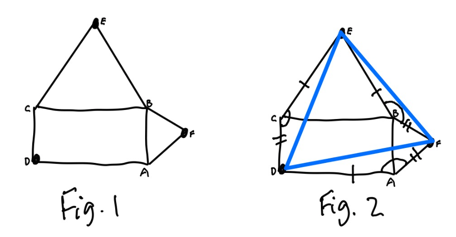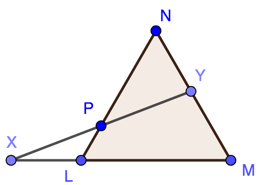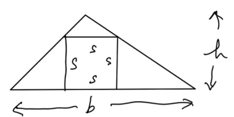## Nice Geometry Problem

Visible to anyone in the world
Edited by Richard Walker, Thursday, 6 Apr 2023, 18:04Suppose we take a rectangle and erect equilateral triangles on two of its side, as shown in Fig. 1. Show that pointsC, E and B are the vertices of an equilateral triangle.

Here is my proof: If we draw in the sides of the triangle (Fig. 2) we can see triangles DCE, BEF and DFA all have

a side of length | and one of length ||
an angle of 150 degrees included between those sides.

Consequently the three triangles are all congruent (the same as on another) and DE = EF = FD, so DEF is equilateral as required.
Share post

## A triangle problem

Visible to anyone in the worldGiven an equilateral triangle LMN, let X lie on ML extended, and Y lie on MN, such that LX = NY.

Show that the point P where XY and LN intersect is the midpoint of XY.

Share post

## Samurai Puzzle - A Square in a Triangle

Visible to anyone in the world
Edited by Richard Walker, Friday, 2 Oct 2020, 09:23

This is a Sangaku-like puzzle (see https://learn1.open.ac.uk/mod/oublog/viewpost.php?post=230691)

We have a square inscribed in a triangle of known base b and height h, as shown. What is the length s of the square's side?I called this a Samurai puzzle because many of the original sangaku were the work of Samurai.